## RS Aggarwal Class 10 Solutions Chapter 16 Co-ordinate Geometry Ex 16A

These Solutions are part of RS Aggarwal Solutions Class 10. Here we have given RS Aggarwal Solutions Class 10 Chapter 16 Co-ordinate Geometry Ex 16A.

Other Exercises

Question 1.
Solution:
(i) A (9, 3) and B (15, 11)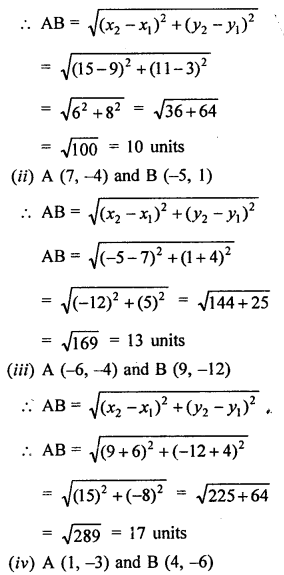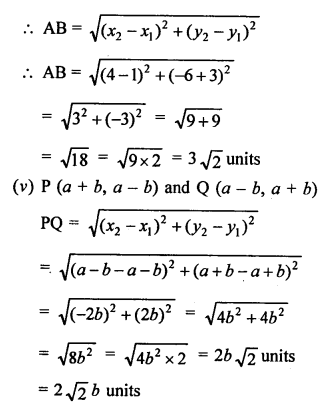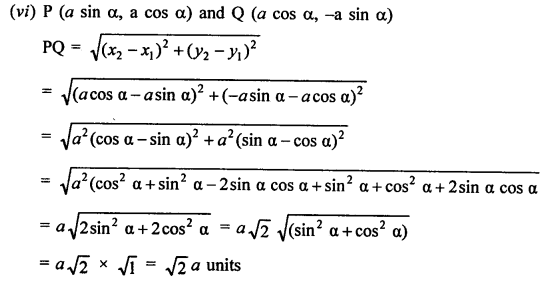Question 2.
Solution:
Distance from origin O (0, 0) and the given points (x, y) = √(x² + y²)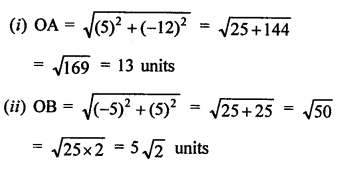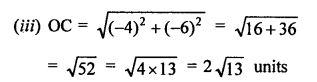Question 3.
Solution:
Points A (x, -1), B (5, 3) and AB = 5 units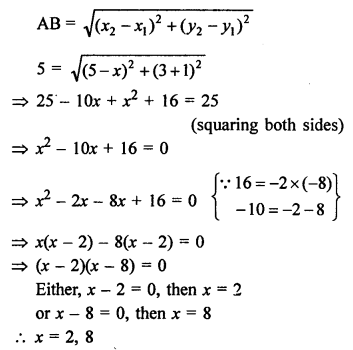Question 4.
Solution:
Points A (2, -3), B (10, y) and AB = 10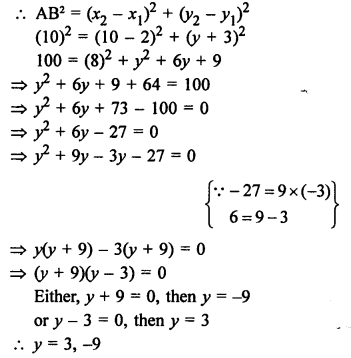Question 5.
Solution:
Points P (x, 4), Q (9, 10) and PQ = 10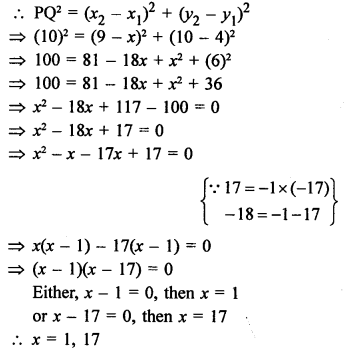Question 6.
Solution:
Point A (x, 2) is equidistant from B (8, -2 and C (2, -2)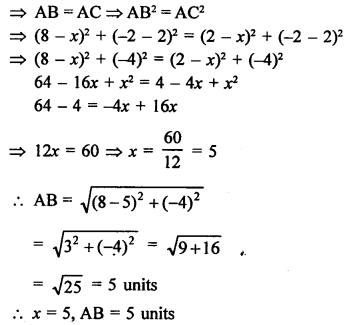Question 7.
Solution:
A (0, 2) is equidistant from B (3, p) and C ip, 5)
Then AB = ACQuestion 8.
Solution:
Let point P (x, 0) is on x-axis and P is equidistant from A (2, -5) and B (-2, 9)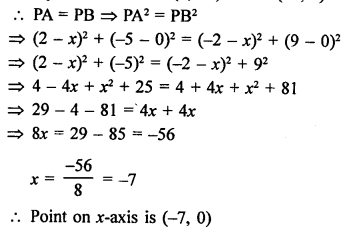Question 9.
Solution:
Let the points on x-axis be P (x,, 0) and Q (x2, 0) and A (11, -8)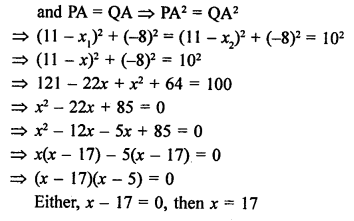or x – 5 = 0, then x = 5
Points are (17, 0) and (5, 0)

Question 10.
Solution:
Let point P (0, y) is on the y-axis, then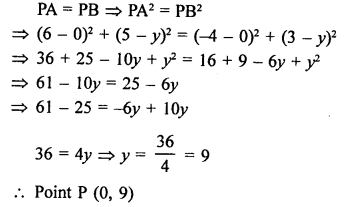Question 11.
Solution:
P (x, y) is equidistant from A (5, 1) and B (-1, 5)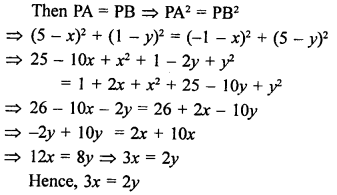Question 12.
Solution:
P (x, y) is equidistant from A (6, -1) and B (2, 3)Question 13.
Solution:
Let the coordinates of the points be O (x, y)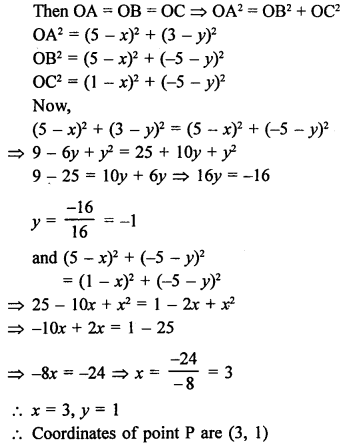Question 14.
Solution:
Points A (4, 3) and B (x, 5) lie on a circle with centre O (2, 3)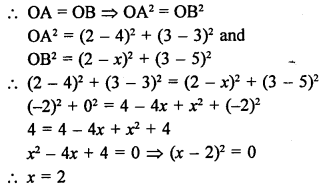Question 15.
Solution:
Point C (-2, 3) is equidistant from points A (3, -1) and B (x, 8)Question 16.
Solution:
Point P (2, 2) is equidistant from the two points A (-2, k) and B (-2k, -3)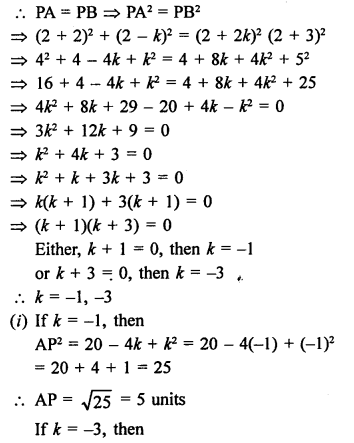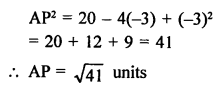Question 17.
Solution:
(i) Let point P (x, y) is equidistant from A (a + b, b – a) and B (a – b, a + b), then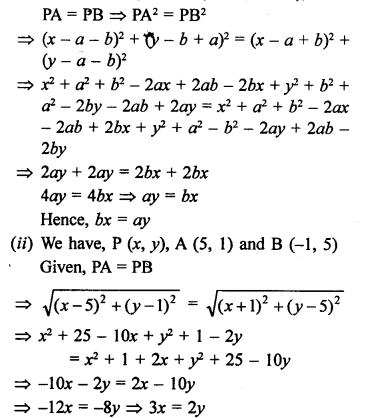Question 18.
Solution:
We know that if the sum of any two of these distances is equal to the distance of the third, then the points are collinear.
Now, (i) Let the points are A (1, -1), B (5, 2), C (9, 5)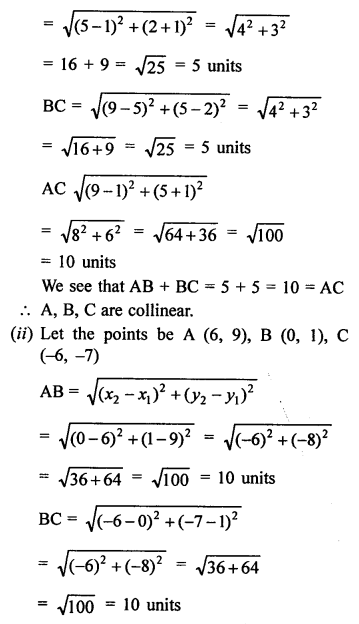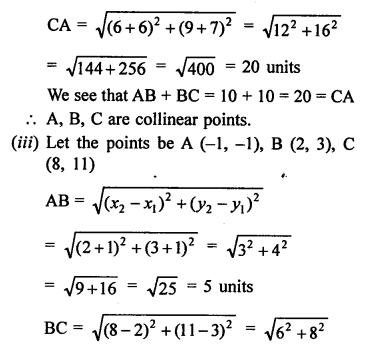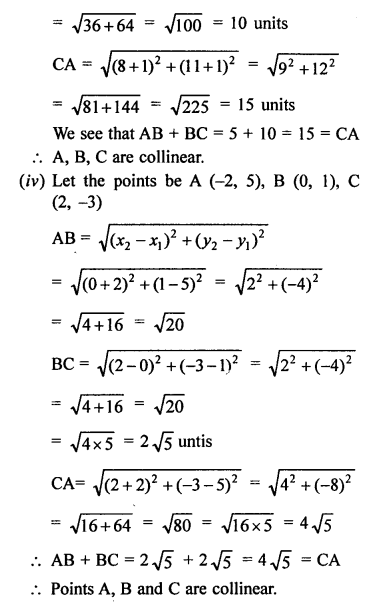Question 19.
Solution:
The points are A (7, 10), B (-2, 5) apd C (3, -4)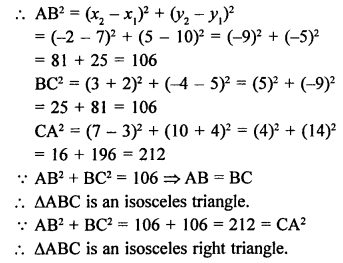Question 20.
Solution:
Points are A (3, 0), B (6, 4) and C (-1, 3)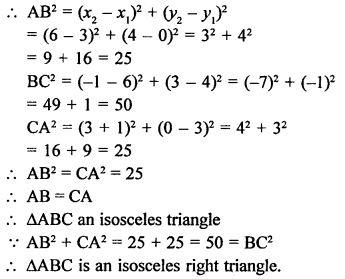Question 21.
Solution:
Points are A (5, 2), B (2, -2) and C (-2, t)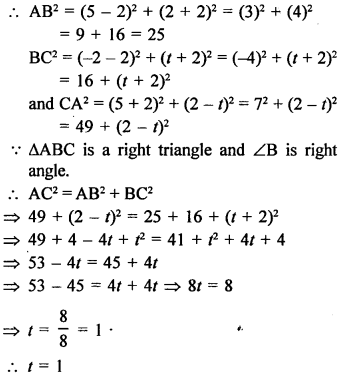Question 22.
Solution:
Points are A (2, 4), B (2, 6) and C (2 + √3, 5)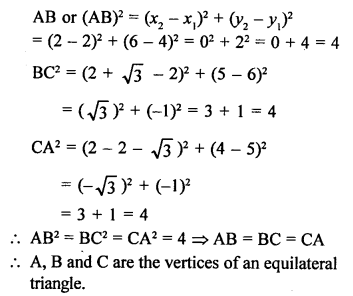Question 23.
Solution:
Let the points are A (-3, -3), B (3, 3), C (-3√3, 3√3)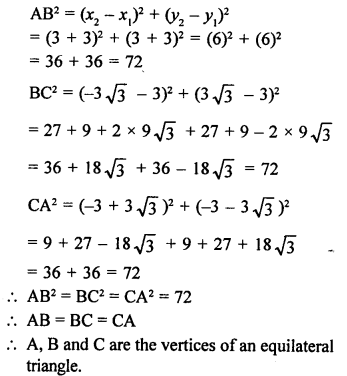Question 24.
Solution:
Points are A (-5, 6), B (3, 0), C (9, 8)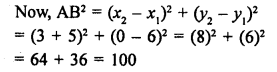Question 25.
Solution:
Points are O (0, 0), A (3, √3) and B (3, -√3)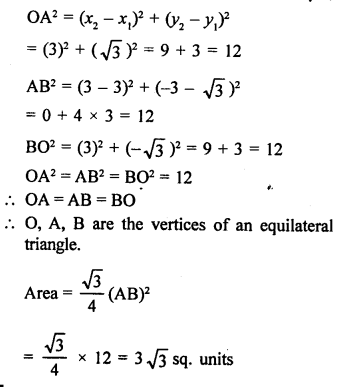Question 26.
Solution:
(i) Points are A (3, 2), B (0, 5), C (-3, 2), D (0, -1)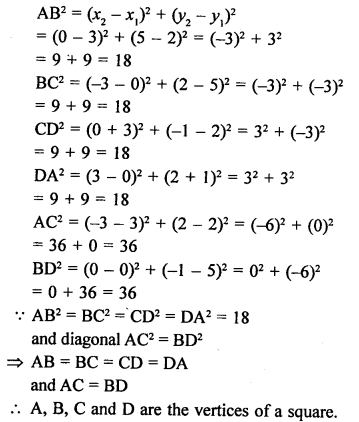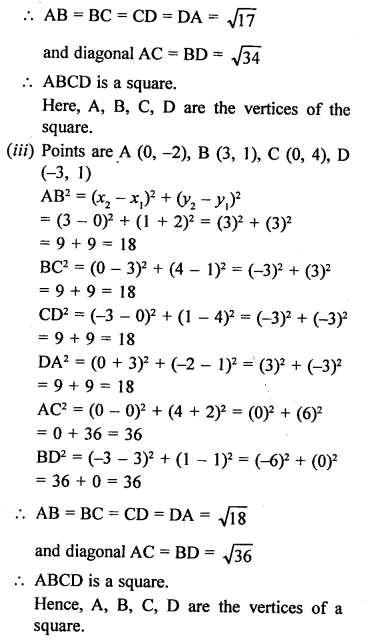Question 27.
Solution:
Points are A (-3, 2), B (-5, -5), C (2, -3), D (4, 4)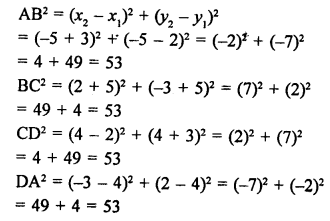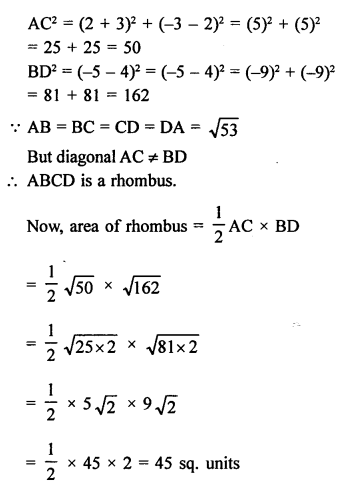Question 28.
Solution:
Points are A (3, 0), B (4, 5), C (-1, 4) and D (-2, -1)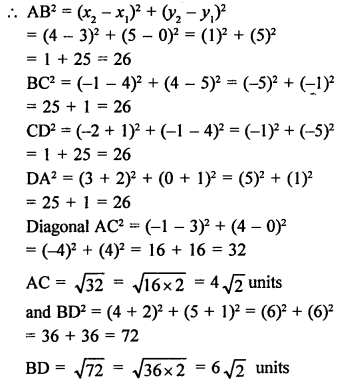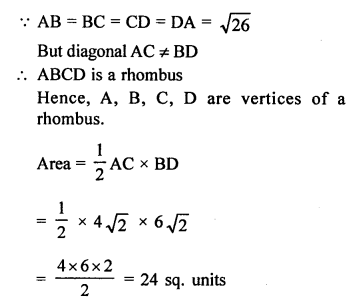Question 29.
Solution:
Points are A (6, 1), B (8, 2), C (9, 4) and D (7, 3)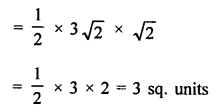Question 30.
Solution:
Points are A (2, 1), B (5, 2), C (6, 4) and D (3, 3)Question 31.
Solution:
Points are A (1, 2), B (4, 3), C (6, 6) and D (3, 5)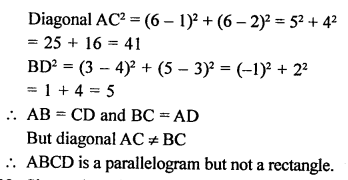Question 32.
Solution:
(i) Points are A (-4, -1), B (-2, -4), C (4, 0) and D (2, 3)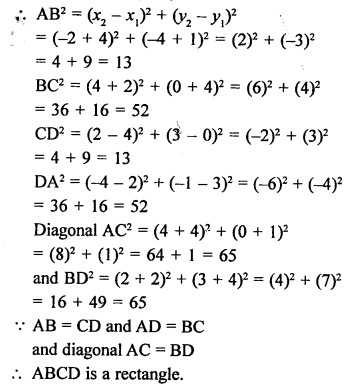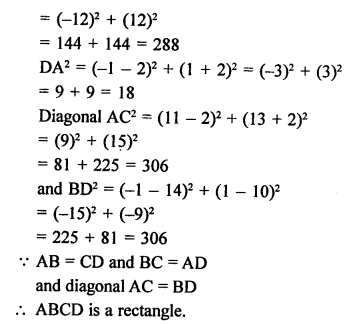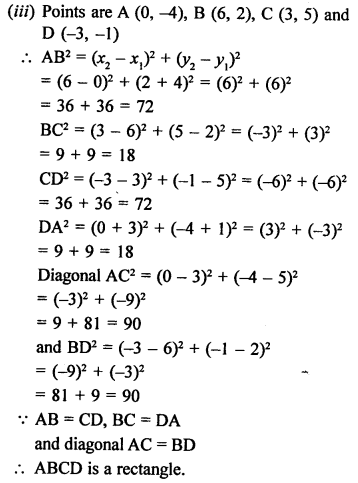Question 33.
Solution: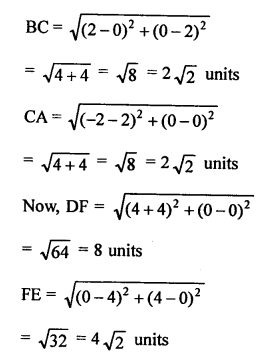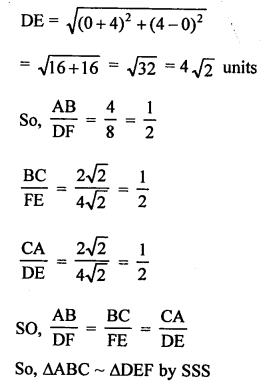Question 34.
Solution: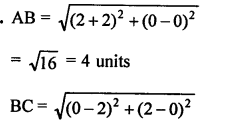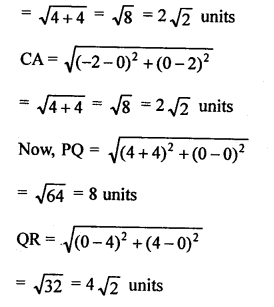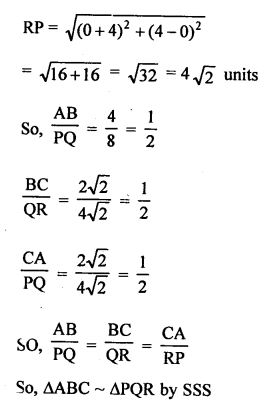Hope given RS Aggarwal Solutions Class 10 Chapter 16 Co-ordinate Geometry Ex 16A are helpful to complete your math homework.

If you have any doubts, please comment below. Learn Insta try to provide online math tutoring for you.